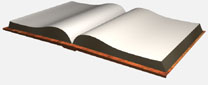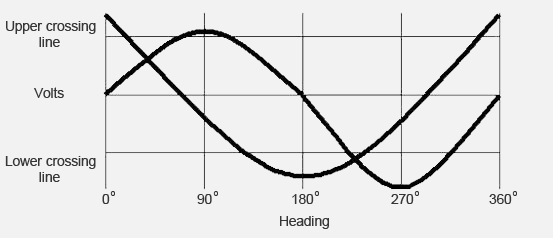# Suggested System For Using An 8-Bit A/D Converter for 1º Accuracy

A sin-cos curve set is plotted on the chart below. If you compare the portions of the curves above and below their crossing voltages, note that above the upper crossing line, the curves are relatively small increments of voltage per degree while below the upper crossing line, the curves are relatively straight and steep. The "crossing line" is drawn at the point where the sin and cos is equal.

Use only the straight and steep portions of either curve for 225 counts on an 8-bit A/D converter, and use the highly curved portions as a sector designator only.When the sin curve is above the upper crossing line, the cos curve, measures from 45° to 135° with a relatively straight line. At 135°, the cos curve (below the lower crossing line) now serves as the designator and to 225°, the sin curve is relatively straight. At 225°, the sin curve is below the lower crossing line and serves as designator so that you can measure a relatively straight portion of the cos curve to 315°. At 315°, the cos curve (above the crossing line) becomes the designator at the upper crossing line, and you have the relatively straight sin curve as the measuring voltage between 315° and 45°.

Previous Page